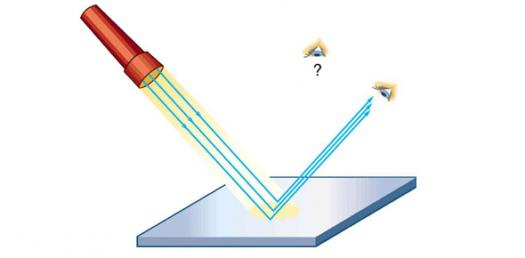# Quiz: Light And Reflection

12 Questions | Attempts: 606
ShareSettingsIn the study of light, something we potentially take for granted every day, reflection is the change in direction of a wavefront at an interface between two different media so that it returns into the medium where it originated.

• 1.
The detection of biological fluids using an ultraviolet light source by forensic investigators is an example of:
• A.

Incandescence

• B.

Fluorescence

• C.

Chemiluminescence

• D.

Bioluminescence

• E.

Phosphorescence

• 2.
In the electromagnetic spectrum, which of the following is TRUE regarding microwaves.
• A.

Their wavelengths are shorter than visible light.

• B.

Microwaves can be detected by human eyes.

• C.

They travel slower than visible light.

• D.

Their wavelenths are longer than visible light.

• E.

They travel faster than visible light.

• 3.
The shortest time interval in which a wave motion completely repeats itself (i.e., makes one complete vibration or oscillation) is called the
• A.

Amplitude

• B.

Wavelength

• C.

Period

• D.

Frequency

• E.

Speed

• 4.
In a transverse wave,
• A.

The particles move parallel to the direction of the wave motion.

• B.

The particles move perpendicular to the direction of the wave motion.

• C.

Energy causes the particles to move forward with the wave.

• D.

Energy is propagated by compressions and rarefactions.

• E.

The speed is unaffected by the type of medium used.

• 5.
The angle of incidence for a wave
• A.

Can be measured between the incident ray and the normal

• B.

Can be measured between the incident wavefront and the boundary

• C.

Is equal to the angle of reflection

• D.

Does not depend on the frequency of the source

• E.

All of the above

• 6.
An student is located 1.8 m from a plane mirror. How far is the image from the student?
• A.

0 m

• B.

0.9 m

• C.

1.8 m

• D.

2.7 m

• E.

3.6 m

• 7.
A concave mirror has a focal length of 30 cm.  If an object is placed 45 from the vertex of this mirror, the image will be:
• A.

Larger and inverted

• B.

Smaller and inverted

• C.

Larger and upright

• D.

Smaller and upright

• E.

The same size and inverted

• 8.
As an object is placed further and further from a concave mirror:
• A.

The image becomes virtual

• B.

The image will get larger and larger

• C.

Eventually, no image will be formed

• D.

The image approaches the focal point

• E.

The image approaches the centre of curvature

• 9.
When using the mirror equation and the magnification equation for curved mirrors, which of the following is FALSE?
• A.

Convex mirrors have a negative focal length

• B.

If an image is larger than the image, its magnification is >1.0

• C.

The distance of the image in convex mirrors will always have a negative value

• D.

The distance of the object is always a positive value, regardless of whether the mirror is concave or convex

• E.

A negative magnification means that the image is inverted

• 10.
An object placed between the focal point and the vertex of a concave mirror will produce:
• A.

No image

• B.

An image that is smaller and virtual

• C.

An image that is larger and virtual

• D.

An image that is smaller and real

• E.

An image that is larger and real

• 11.
All parallel rays that approach a concave mirror:
• A.

Must reflect through the focal point

• B.

Must reflect through the centre of curvature

• C.

Must reflect somewhere between the focal point and the centre of curvature

• D.

Must reflect back along the incident path

• E.

None of the above

• 12.
All images in plane mirrors are:
• A.

The same size as the object and real

• B.

Larger and upright

• C.

Smaller and upright

• D.

Smaller and inverted

• E.

Virtual and upright

## Related TopicsBack to top
×

Wait!
Here's an interesting quiz for you.